×#### Thank you for registering.

One of our academic counsellors will contact you within 1 working day.

Click to Chat

1800-1023-196

+91-120-4616500

CART 0

• 0

MY CART (5)

Use Coupon: CART20 and get 20% off on all online Study Material

ITEM
DETAILS
MRP
DISCOUNT
FINAL PRICE
Total Price: Rs.

There are no items in this cart.
Continue Shopping• Complete JEE Main/Advanced Course and Test Series
• OFFERED PRICE: Rs. 15,900
• View Details

```Revision Notes on Straight Lines

Given two points P(x1, y1) and Q(x2, y2) the length PQ is given by Length PQ = √(x2 – x1)2 + (y2 – y1)2.

Section Formula:

Given two points A(x1, y1) and B(x2, y2), the coordinates of the point which divide the line segment AB in the ratio m: n are [(nx1 + mx2)/(m + n), (ny1 + my2)/(m + n)] (For internal division)

Given two points A(x1, y1) and B(x2, y2), the coordinates of the point which divide the line segment AB in the ratio m: n are [(mx2  - nx1 )/(m+n), ( my2 -ny1)/(m+n)] (For external division)

The ratio m:n can also be written as m/n or λ:1. So any point on line joining A and B will be P((λx2 + x1)/(λ + 1), (λy2 + y1)/(λ + 1)).

If two points P and Q divide the line AB internally and externally in the same ratio m:n, then P and Q are said to be harmonic conjugate of each other with respect to A and B.

The centroid G of the triangle ABC, divides the median AD, in the ratio of 2 : 1.

If the vertices of the triangle ABC are A(x1, y1), B(x2, y2), C(x3, y3), then the coordinates of the incentre are given by x = (ax1 + bx2 + cx3)/(a + b + c), y = (ay1 + by2 + cy3)/(a + b + c).

Excentre of a triangle is the point of concurrency of bisectors of two exterior and the third interior angle. Hence, a triangle has three excentres one opposite to each of its vertex.

Consider a triangle ABC with vertices A(x1, y1), B(x2, y2) and C(x3, y3). As shown in the figure below, I1, I2 and I3 are the centres of the ex-circles opposite to the vertices A, B and C respectively.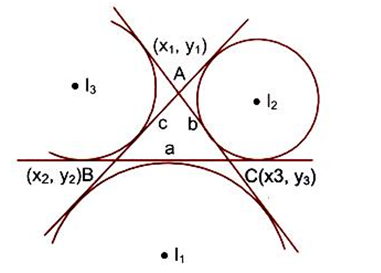The coordinates of I1, I2 and I3 are given by

I1(x, y) = (–ax1 + bx2 + cx3)/(a + b + c) ,(–ay1 +  by2 + cy3) / (–a + b + c)

I2(x, y) = (ax1 – bx2 + cx3)/(a – b + c), (ay1 – by2 + cy3)/(a – b + c)

I3(x, y) = (ax1 + bx2 – cx3)/(a + b – c), (ay1+ by2 – cy3)/(a + b – c)

Area of a polygon of n sides:

?Plot the points and check their actual order.

Let A1(x1, y1), A2 (x2, y2), ….…,An(xn, yn) be the vertices of the polygon taken in anticlockwise direction.

Area of the polygon is half the value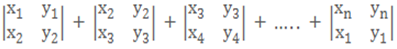Area of a triangle can also be expressed as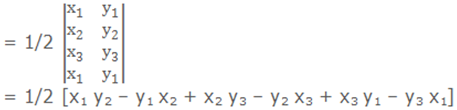The area of a triangle is positive when the vertices are taken in the anticlockwise direction and negative when the vertices are taken in clockwise direction.

The area of the triangle formed by three collinear points P1, P2 and P3 is zero i.e. their determinant must vanish.

If θ is the angle at which a straight line is inclined to the positive direction of the x-axis, then m = tan θ, (0 < θ < 180o) is the slope of the line.

Equations of straight line in various forms:

Slope Intercept From: y = mx + c, where m = slope of the line and c is the y intercept

Intercept Form: x/a + y/b = 1, where x intercept = a and y intercept = b

Slope point form: y – y1 = m(x – x1), where (x1, y1) is a point on the straight line and m is the slope.

Two points form: Consider two points A(x1, y1) and B(x2, y2) in a co-ordinate plane. If any point P(x, y) lies on the line joining A and b then m = tan θ = (y–y1)/(x–x1) = (y2 – y1)/(x2 – x1)

Parametric form: Consider line PQ with points Q(x1, y1). Then Co-ordinates of any points P(x, y) are x = x1 + r cos θ and y = y1 + r sin θ
Then the equation of the line is obtained as ⇒ (x–x1)/cos θ = (y–y1)/sin θ = r

Normal form: Consider line l as shown in figure given belowA and B are intercept points of line l. So intercepts on x and y-axes are p/cos α and p/sin α respectively. The equation of line l is x cos α/p + y sin α/p = 1

⇒ xcos α + y sin α = p, where p is the perpendicular distance from the origin.

Angle bisector of two lines is the locus of a point which is equidistant (having equal perpendicular distance) from the two lines.

Given two lines L1 : A1x + B1y + C1 = 0 and L2 : A2x + B2y + C2 = 0, if point R(p, q) lies on the bisector, then length of perpendicular from P to be both lines should be equal and hence the equation of the angle bisector is given as (A1x+B1y+C1)/√A12 + B12 = + (A2x+B2y+C2) /√A22 + B22.

The above equation yields two bisectors, one acute angle bisector and the other obtuse bisector.

How to decide a particular bisector:

Consider two lines L1: A1x + B1y + C1 = 0 and L2: A2x + B2y + C2 = 0. To determine a bisector which lies in the same relative position with respect to the lines as a given point S(x3, y3) does, make the signs of the expressions A2x3 + B1y3 + C1 and A2x3 + B2y3 + C2 identical. (say positive)

Now, (A1x + B1y + C1)/√A12 + B12 = + (A2x +  B2y + C2)/√A22 + B22 gives the bisector towards this point.

If the signs are different multiply one of the equations with ‘–1’ throughout, so that positive sign is obtained. Then above equation with changed equations of lines will given the required bisector.

If (x3, y3) ≡ (0, 0) and A2A1 + B2B1 > 0 then the bisector towards the origin is the obtuse angle bisector.

How to detect whether the bisector is of the acute or obtuse angle:

First determine both the bisectors.

Then, calculate the angle between one of them and the initial line.

The bisector for which |tan θ| > 1 is the obtuse angle bisector.

The equation to the locus is the relation which exists between the coordinates of all the points on the path, and which holds for no other points except those lying on the path.

How to find the equation of the locus of a point:

If we are finding the equation of the locus of a point P, assign coordinates (h, k) to P.

Try to state the given conditions as equations in terms of the known quantities and unknown parameters.

Eliminate the parameters, so that you are left with only h, k and known quantities.

Replace h by x, and k by y, in the obtained equation. The resulting equation is the equation of the locus of p.

If the two lines are perpendicular to each other then m1m2 = –1, i.e. the product of their slopes is -1.

Any line perpendicular to ax + by + c = 0 is of the form bx – ay + k = 0.

If the two lines are parallel or are coincident, then m1 = m2.

Any line parallel to ax + by + c = 0 is of the form ax + by + k = 0.

Let there be two-lines l1 and l2 with slopes m1 and m2 respectively. So tan α = m1, tan β = m2. Angle between them is either α – β or π – (α – β) depending on the side taken into consideration.

The lines a1x + b1y + c1 = 0            …… (i)?

and a2x + b2y + c2 = 0                    …… (ii) represent

intersecting lines if a1/a2 ≠ b1/b2

parallel lines if a1/a2 = b1/b2

Coincident lines if a1/a2 = b1/b2 = c1/c2

The normal equation can be used to find the distance of a point from a straight line.

To find the distance of the point P(x1, y1) from the line l1 whose equation is x cos α + y sin α = p:

Let l2 be the line through P parallel to the line l1. Let d be the distance of P from l1.

Then, the normal from O to l2 is of length p + d. Hence the equation of l2 isx cos α + y sin α = p + d.

Since P(x1, y1) lies on it, so it must satisfy the equation ∴ x1 cos α + y1 sin α = p + d
∴ d = x1 cos α + y1 sin α – p.

Complete Distance Formula: If the point P and the origin O lie on the same side of a line as shown in the figure given below, then d = – (x1 cos α + y1 sin α – p).
Hence, the complete distance formula is d = + (x1 cos α + y1 sin α – p). This gives d = + (ax1 + by1 + c)/√a2 + b2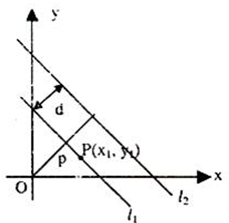To find the distance of a point from the given line, in the left side of the equation (right side being zero) substitute the co-ordinates of the point, and divide the result by √(coefficient of x)2 + (coefficient of y)2.

The complete perpendicular distance formula is used when the length of the perpendicular to the line is given.

Consider two parallel lines y = mx + c1 and y = mx + c2. Then to find the distance between these two lines:

The line y = mx + c1 intersects the x-axis at the point A (–c1/m, 0).

The distance between these two lines is equal to the length of the perpendicular from point A to line (2). Therefore, distance between these lines is given by
|(–m)(–c1/m) + (–c2)|/√1+m2 or d = |c1–c2|/√1+m2.
Thus the distance d between two parallel lines y = mx + c1 and y + mx + c2 is given by d = |c1–c2|/√A2 + B2.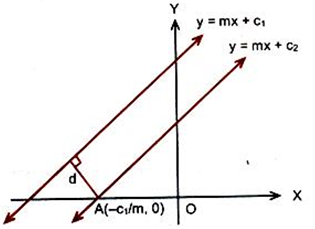.

The general equation of the family of lines through the point of intersection of two given lines is L + λL’ = 0, where L = 0 and L’ = 0 are the two given lines, and λ is a parameter.

To find the straight line equations which pass through a given point (x1, y1) and make equal angles with the given straight line y = m1x + c:

If m is the slope of the required line and α is the angle which this line makes with the given line, then tanα = + (m1–m)/(1+m1m).

The above expression for tanα, gives two values of m, say mA and mB.

The required equations of the lines through the point (x1, y1) and making equal angles α with the given line are

y – y1= mA (x – x1), y – y1= mB(x – x1).

The condition for three lines to be concurrent:

Let the three given lines be a1x + b1y + c1 = 0, a2x + b2y + c2 = 0 and a3x + b3y + c3 = 0.?????

ThenThere exist 3 constants l, m, n (not all zero at the same time) such that IL1 + mL2 + nL3 = 0, where L1 = 0, L2 = 0 and L3 = 0 are the three given straight lines.

Consider a line ax + by + c = 0 and P(x1, y1), Q(x2, y2) be two points.

If P(x1, y1) and Q(x2, y2) are on the opposite sides of the line ax + by + c = 0, then the point R on the line ax + by + c = 0 divides the line PQ internally in the ratio m1: m2, where m1/m2 must be positive.

Co-ordinates of R are (m1x2 + m2x1/m1+m2, m1y2 + m2y1/m1+m2).

Point R lies on the line ax + by + c = 0
⇒ m1/m2 = (ax1+by1+c)/(ax2+by2+c ) > 0

If ax1 + by1 + c and ax2 + by2 + c have the same signs then m1/m2 = –ve, so that the point R on the line ax + by + c = 0 will divide the line PQ externally in the ratio m1: m2 and the points P(x1, y1) and Q(x2, y2) are on the same side of the line ax + by + c = 0.

If we have two lines with equations a1x + b1y+ c1 = 0 and a2x + b2y + c2 = 0, then the equations of the bisectors are given by (a1x + b1y + c1)/√a12 + b12 = + (a2x + b2y + c2)/√a22 + b22

If c1 and c2 are of same sign, then evaluate a1a2 + b1b2. If this value is negative, then the acute angle bisector is (a1x+b1y+c1)/√a12+b12 = + (a2x+b2y+c2)/√a22+b22.

If both c1 and c2 are of the same sign, in that case also, the equation of the angle bisector which contains the origin is the same as the previous case.

Homogeneous equation of degree two of the form ax2 + 2hxy + by2 = 0 represents a pair of straight lines passing through the origin, provided the following conditions hold good:

If h2 > ab then the lines are real and distinct.

If h2 = ab, the lines are coincident

If h2 < ab, then the lines are imaginary with real point of intersection i.e. (0, 0).

If y = m1x and y = m2x are two equations characterized by ax2 + 2hxy + by2 = 0, then the two values of y are like solutions of the equation and hence, m1 + m2 = -2h/ b and m1m2 = a/b.

The equation ax2 + 2hxy + by2 + 2gx + 2fy + c = 0 symbolizes a straight line if it satisfies the condition –
abc + 2fgh –af2 – bg2 – ch2 = 0.

The equation of the straight line bisecting the angle between the straight lines ax2 + 2hxy + by2 = 0 is (x2 – y2)/ (a-b) = xy / h.

Any second degree curve passing through the four points of intersection of f(xy) = 0 and xy = 0 is given by the relation f(xy) + μxy = 0, where f(xy) = 0 is also a second degree curve.

```### Course Features

• 728 Video Lectures
• Revision Notes
• Previous Year Papers
• Mind Map
• Study Planner
• NCERT Solutions
• Discussion Forum
• Test paper with Video Solution